MCAT Biology : Population Genetics and Hardy-Weinberg

Example Questions

1 2 4 Next →

Example Question #5 : Mendelian And Population Genetics

A rare recessive mutation causes rabbits that are normally white to be pink. If one out of every 625 rabbits is pink, what percentage of the population is heterozygous?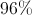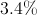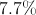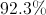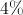Explanation:

We can use the Hardy-Weinberg equilibrium formulas to calculate the allele frequencies.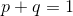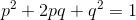We know that the frequency of homozygous recessive (pink) rabbits is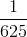. This is equal to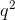in the Hardy-Weinberg calculation. We can use this information to solve for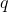, the recessive allele frequency.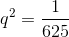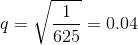Now that we know the value of, we can solve for the value of.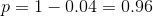The frequency of heterozygotes is equal to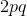in the Hardy-Weinberg calculation. Now that we know the frequency of each allele, we can complete this calculation.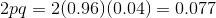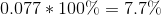1 2 4 Next →